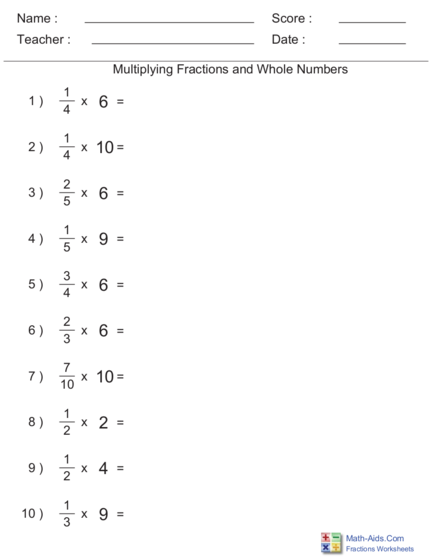# Multiplying Fractions by Whole Numbers worksheetContributed by:Multiplying Fractions by Whole Numbers worksheet.
Write the whole number as a fraction with a denominator of 1.
Multiply the numerators.
Multiply the denominators.
1. Name : Score :
Teacher : Date :
Multiplying Fractions and Whole Numbers
1
1)
4
x 6 =
1
2)
4
x 10 =
2
3)
5
x 6 =
1
4)
5
x 9 =
3
5)
4
x 6 =
2
6)
3
x 6 =
7
7)
10
x 10 =
1
8)
2
x 2 =
1
9)
2
x 4 =
1
10 )
3
x 9 =
Math-Aids.Com
Fractions Worksheets
2. Name : Score :
Teacher : Date :
Multiplying Fractions and Whole Numbers
1 1 x 6 6 3 1
1)
4
x 6 =
4 x 1
=
4
=
2
= 1 2
1 1 x 10 10 5 1
2)
4
x 10 = 4 x 1
=
4
=
2
= 2 2
2 2 x 6 12 2
3)
5
x 6 =
5 x 1
=
5
= 2 5
1 1 x 9 9 4
4)
5
x 9 =
5 x 1
=
5
= 1 5
3 3 x 6 18 9 1
5)
4
x 6 =
4 x 1
=
4
=
2
= 4 2
2 2 x 6 12 4
6)
3
x 6 =
3 x 1
=
3
=
1
= 4
7 7 x 10 70 7
7)
10
x 10 = 10 x 1
=
10
=
1
= 7
1 1 x 2 2
8)
2
x 2 =
2 x 1
=
2
= 1 = 7
1 1 x 4 4 2
9)
2
x 4 =
2 x 1
=
2
=
1
= 2
1 1 x 9 9 3
10 )
3
x 9 =
3 x 1
=
3
=
1
= 3
Math-Aids.Com
Fractions Worksheets